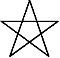There are eight months in the Calendar of Mu, sometimes referred to as the Nyarzirian Calendar. Each month consists of forty-five days, and represents a system of the eight hexagrams moving through the five elements. The Ninzuwu Priest/Priestess invokes the mudra/mantra formulae associated with each hexagram that rules a particular day after invoking the Armor of Amaterasu Ohkami. The chart below illustrates the daily Vasuh letters invoked and the ruling “deity” for the said hexagram:
1. December 25th 2014 = Midzuhanome = 3rd Hexagram = Shki-Zhee
2. December 26th 2014 = Susanoo = 5th Hexagram = Phe-Tuu-Nzu
3. December 27th 2014 = Nudzuwata = 8th Hexagram = Tuu-Zhee
4. December 28th 2014 = Watatsumi = 29th Hexagram = Aum-Shki
5. December 29th 2014 = Ugajin = 39th Hexagram = Phe-Bnhu-Shki
6. December 30th 2014 = Ame-no-Uzume = 48th Hexagram = Hmu-Shki-Aum
7. December 31st 2014 = Izanami-no-Mikoto = 60th Hexagram = Nzu-Phe-Shki
8. January 1st 2015 = Ajisukitakahikone = 63rd Hexagram = Aum-Nzu
9. January 2nd 2015 =Entering Earth Element
10. January 3rd 2015 = Midzuhanome = 3rd Hexagram = Shki-Zhee
11. January 4th 2015 = Susanoo = 5th Hexagram = Phe-Tuu-Nzu
12. January 5th 2015 = Nudzuwata = 8th Hexagram = Tuu-Zhee
13. January 6th 2015 = Watatsumi = 29th Hexagram = Aum-Shki
14. January 7th 2015 = Ugajin = 39th Hexagram = Phe-Bnhu-Shki
15. January 8th 2015 = Ame-no-Uzume = 48th Hexagram = Hmu-Shki-Aum
16. January 9th 2015 = Izanami-no-Mikoto = 60th Hexagram = Nzu-Phe-Shki
17. January 10th 2015 = Ajisukitakahikone = 63rd Hexagram = Aum-Nzu
18. January 11th 2015 =Entering Water Element
19. January 12th 2015 = Midzuhanome = 3rd Hexagram = Shki-Zhee
20. January 13th2015 = Susanoo = 5th Hexagram = Phe-Tuu-Nzu
21. January 14th2015 = Nudzuwata = 8th Hexagram = Tuu-Zhee
22. January 15th2015 = Watatsumi = 29th Hexagram = Aum-Shki
23. January 16th2015 = Ugajin = 39th Hexagram = Phe-Bnhu-Shki
24. January 17th2015 = Ame-no-Uzume = 48th Hexagram = Hmu-Shki-Aum
25. January 18th2015 = Izanami-no-Mikoto = 60th Hexagram = Nzu-Phe-Shki
26. January 19th2015 = Ajisukitakahikone = 63rd Hexagram = Aum-Nzu
27. January 20th2015 =Entering Fire Element
28. January 21st 2015 = Midzuhanome = 3rd Hexagram = Shki-Zhee
29. January 22nd2015 = Susanoo = 5th Hexagram = Phe-Tuu-Nzu
30. January 23rd 2015 = Nudzuwata = 8th Hexagram = Tuu-Zhee
31. January 24th 2015 = Watatsumi = 29th Hexagram = Aum-Shki
32. January 25th 2015 = Ugajin = 39th Hexagram = Phe-Bnhu-Shki
33. January 26th 2015 = Ame-no-Uzume = 48th Hexagram = Hmu-Shki-Aum
34. January 27th 2015 = Izanami-no-Mikoto = 60th Hexagram = Nzu-Phe-Shki
35. January 28th 2015 = Ajisukitakahikone = 63rd Hexagram = Aum-Nzu
36. January 29th 2015 =Entering Air Element
37. January 30th 2015 = Midzuhanome = 3rd Hexagram = Shki-Zhee
38. January 31st 2015 = Susanoo = 5th Hexagram = Phe-Tuu-Nzu
39. February 1st 2015 = Nudzuwata = 8th Hexagram = Tuu-Zhee
40. February 2nd 2015 = Watatsumi = 29th Hexagram = Aum-Shki
41. February 3rd 2015 = Ugajin = 39th Hexagram = Phe-Bnhu-Shki
42. February 4th 2015 = Ame-no-Uzume = 48th Hexagram = Hmu-Shki-Aum
43. February 5th 2015 = Izanami-no-Mikoto = 60th Hexagram = Nzu-Phe-Shki
44. February 6th 2015 = Ajisukitakahikone = 63rd Hexagram = Aum-Nzu
45. February 7th 2015 =Entering Void Element